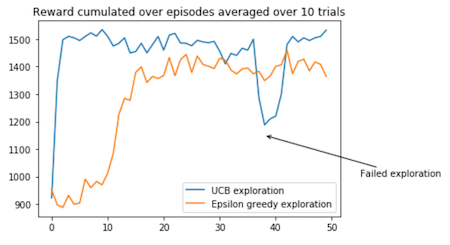@王小新 王小新 编译自 Medium

Q-Learning是强化学习中最常用的算法之一。

Medium上有篇文章，讨论了这种算法的一个重要部分：搜索策略。

## 马尔可夫决策过程

Markov decision process

Markov Decision Process (MDP) Toolbox:

• S是状态空间（有限），包括3种不同年龄树木，年龄级分别为0-20年、21-40年和40年以上；
• A是动作空间（有限），即等待或砍伐；
• P和R分别是转移矩阵和奖励矩阵，很容易写出它的闭合形式；
• γ是数值在0与1之间的贴现因子，用来平衡短时和未来奖励的关系；
• 策略π是当前状态下决策的静态分布；

`````` 1def optimal_value_iteration(mdp, V0, num_iterations, epsilon=0.0001):
2    V = np.zeros((num_iterations+1, mdp.S))
3    V[:] = np.ones(mdp.S)*V0
4    X = np.zeros((num_iterations+1, mdp.A, mdp.S))
5    star = np.zeros((num_iterations+1,mdp.S))
6    for k in range(num_iterations):
7        for s in range(mdp.S):
8            for a in range(mdp.A):
9                X[k+1][a][s] = mdp.R[a][s] + mdp.discount*np.sum(mdp.P[a][s].dot(V[k]))
10            star[k+1][s] = (np.argmax(X[k+1,:,s]))
11            V[k+1][s] = np.max(X[k+1,:,s])
12        if (np.max(V[k+1]-V[k])-np.min(V[k+1]-V[k])) < epsilon:
13            V[k+1:] = V[k+1]
14            star[k+1:] = star[k+1]
15            X[k+1:] = X[k+1]
16            break
17        else: pass
18    return star, V, X

``````

## Q-Learning算法

Q-Learning算法中的“Q”代表着策略π的质量函数（Quality function），该函数能在观察状态s确定动作a后，把每个状态动作对 (s, a) 与总期望的折扣未来奖励进行映射。

Q-Learning算法属于model-free型，这意味着它不会对MDP动态知识进行建模，而是直接估计每个状态下每个动作的Q值。然后，通过在每个状态下选择具有最高Q值的动作，来绘制相应的策略。

http://v.qq.com/x/page/i0658azj9ut.htmlv.qq.com

`````` 1def q_learning(mdp, num_episodes, T_max, epsilon=0.01):
2    Q = np.zeros((mdp.S, mdp.A))
3    episode_rewards = np.zeros(num_episodes)
4    policy = np.ones(mdp.S)
5    V = np.zeros((num_episodes, mdp.S))
6    N = np.zeros((mdp.S, mdp.A))
7    for i_episode in range(num_episodes):
8        # epsilon greedy exploration
9        greedy_probs = epsilon_greedy_exploration(Q, epsilon, mdp.A)
10        state = np.random.choice(np.arange(mdp.S))
11        for t in range(T_max):
12            # epsilon greedy exploration
13            action_probs = greedy_probs(state)
14            action = np.random.choice(np.arange(len(action_probs)), p=action_probs)
15            next_state, reward = playtransition(mdp, state, action)
16            episode_rewards[i_episode] += reward
17            N[state, action] += 1
18            alpha = 1/(t+1)**0.8
19            best_next_action = np.argmax(Q[next_state])
20            td_target = reward + mdp.discount * Q[next_state][best_next_action]
21            td_delta = td_target - Q[state][action]
22            Q[state][action] += alpha * td_delta
23            state = next_state
24        V[i_episode,:] = Q.max(axis=1)
25        policy = Q.argmax(axis=1)
26    return V, policy, episode_rewards, N``````

## 探索与利用的平衡

• 利用：根据当前信息做出最佳决策；
• 探索：做出其他决策来收集更多信息。

## ε-greedy搜索策略

``````1def epsilon_greedy_exploration(Q, epsilon, num_actions):
2    def policy_exp(state):
3        probs = np.ones(num_actions, dtype=float) * epsilon / num_actions
4        best_action = np.argmax(Q[state])
5        probs[best_action] += (1.0 - epsilon)
6        return probs
7    return policy_exp

``````

## 不确定优先搜索策略

• 利用：在给定状态下选择具有最高Q值的动作；
• 探索：做出其他决策来探索更多信息，通过选择不了解或不够了解的环境。

• 若当前处于最佳环境，那算法会直接选择最佳的手臂；
• 若当前不处于最佳环境，则算法会尽量降低不确定性。

• Q(s, a)：状态s下动作a缩放后的Q值；
• N(t，s，a)：在时刻t和状态s下动作a被选择的次数。

(Q(s,a) − )为相应误差项。

``````1def UCB_exploration(Q, num_actions, beta=1):
2    def UCB_exp(state, N, t):
3        probs = np.zeros(num_actions, dtype=float)
4        Q_ = Q[state,:]/max(Q[state,:]) + np.sqrt(beta*np.log(t+1)/(2*N[state]))
5        best_action = Q_.argmax()
6        probs[best_action] = 1
7        return probs
8    return UCB_exp

``````

UCB搜索算法应该能很快地获得高额奖励，但是前期搜索对训练过程的影响较大，有希望用来解决更复杂的多臂赌博机问题，因为这种方法能帮助智能体跳出局部最优值。

## 总结与展望

Q-Learning是强化学习中最常用的算法之一。在这篇文章中，我们讨论了搜索策略的重要性和如何用UCB搜索策略来替代经典的ε-greedy搜索算法。

## 参考文献

 T. Jaakkola, M. I. Jordan, and S. P. Singh, “On the convergence of stochastic iterative dynamic programming algorithms” Neural computation, vol. 6, no. 6, pp. 1185–1201, 1994.

 P. Auer, “Using Confidence Bounds for Exploitation-Exploration Trade-offs”, Journal of Machine Learning Research 3 397–422, 2002.

 E. Even-Dar, and Y. Mansour, “Learning Rates for Q-learning”, Journal of Machine Learning Research 5 1–25, 2003.

 A. Baransi, O.-A. Maillard, and S. Mannor, “Sub-sampling for multi-armed bandits”, Joint European Conference on Machine Learning and Knowledge Discovery in Databases, 115–131, 2014.

վ'ᴗ' ի 追踪AI技术和产品新动态